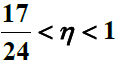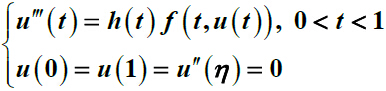# 一类奇异三阶三点边值问题正解的存在性Positive Solutions for a Third-Order Three-Point Singular Boundary Value Problem

DOI: 10.12677/AAM.2016.51004, PDF, HTML, XML, 下载: 1,467  浏览: 3,268  国家自然科学基金支持

Abstract: 其中，非线性项允许在t=0, t=1及u=0 处奇异，利用锥上的不动点定理在较弱的条件下得到了上述边值问题至少存在一个、两个、n个正解的存在性结果。 This paper is concerned with the following nonlinear third-order three-point boundary value problemwhere, the nonlinear term may be singular at t=0, t=1  and u=0 . By using fixed- point theorem in cone, the existence of one or two or n positive solutions is obtained with the weaker conditions.

  Gregus, M. (1987) Third Order Linear Differential Equations. Reidel, Dordrecht. http://dx.doi.org/10.1007/978-94-009-3715-4  Anderson, D.R. (2003) Green’s Function for a Third-Order Generalized Right Focal Problem. Journal of Mathematical Analysis and Applications, 288, 1-14. http://dx.doi.org/10.1016/S0022-247X(03)00132-X  Sun, Y.P. (2005) Positive Solutions of Singular Third-Order Three-Point Boundary Value Problem. Journal of Mathematical Analysis and Applications, 306, 589-603. http://dx.doi.org/10.1016/j.jmaa.2004.10.029  Sun, Y.P. (2009) Positive Solutions for Third-Order Three-Point Nonhomogeneous Boundary Value Problem. Applied Mathematics Letters, 22, 45-51. http://dx.doi.org/10.1016/j.aml.2008.02.002  Anderson, D.R. and Avery, R. (2001) Multiple Positive Solutions to a Third-Order Discrete Flocal Boundary Value Problems. Computers & Mathematics with Applications, 42, 333-340. http://dx.doi.org/10.1016/S0898-1221(01)00158-4  Kaufmann, E.R. (2003) Positive Solutions of a Three-Point Boundary Value Problem on a Time Scale. Electron. J. Diff. Equa., 82, 1-11.  Anderson, D.R., Anderson, T. and Kleber, M. (2006) Green’s Function and Existence of Soluyions for a Functional Focal Differential Equations. Electron. J. Diff. Equa., 12, 1-14.  Palamides, A.P. and Smyrlis, G. (2008) Positive Solutions to a Singular Third-Order Three-Point Boundary Value Problem with an Indefinitely Signed Green’s Function. Nonlinear Analysis, 68, 2104-2118. http://dx.doi.org/10.1016/j.na.2007.01.045  Wu, H.P. (2011) Multiple Positive Solutions to a Singular Third-Order Three-Point Boundary Value Problem. Annals of Differential Equations, 27, 379-383.  Guo, D. and Lakshmikantham, V. (1988) Nonlinear Problems in Abstract Cone. Academic Press, San Diego.